## Monday, May 31, 2010

### Roughing and Sawing in the mill

I am making some brass parts on my mill that require some drilling, milling, and sawing with a jeweler's saw. I thought that this would be a good chance to try HeeksCNC out with these operations.
HeeksCNC has some very good operations for drilling and profiling that would be handy for this, but there is nothing in the way of automatic roughing or multi pass sawing. I ended up using some other CAD tricks and HeeksCNC's python scripting to make things work.
Here is the drawing as it imported into HeeksCAD: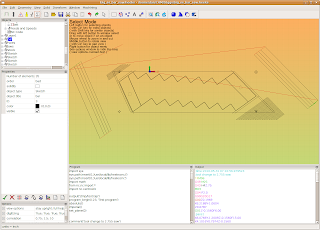The stair step shaped part is the thing that I am ultimately cutting out. On the right and left corners of it, I have added (in another CAD program) some roughing passes to cut the corners of my stock off:The roughing actually looks like this on my Bridgeport mill:I also needed to saw some slits in the sides of the part. I wanted to do the sawing with 4 passes of the saw blade on each side. I used my CAD program to offset the final saw path back half the diameter of the saw blade and then offset it 3 additional times by .050".Obviously, it's critical not to retract the saw blade in the Z axes at the wrong time,and I wanted to keep the saw blade down at the right Z level while doing these passes, so I needed to do something to avoid using HeeksCNC's usual profiling method of doing a path, retracting in Z and then doing the next path. I resorted to some Python scripting tricks.
1. I selected the lines that I had drawn in CAD for sawing in HeeksCNC and generated a Profile operation with them. This allowed me to extract the geometric points that I needed.
2. I copied the python script from HeeksCNC and pasted it into my favorite text editor.
3. The script was easy to understand and manipulate- I just wanted a series of 'feed' and 'rapid' in the right spots.I didn't use any loops, because I was lazy....

Here is my sawing script:

comment('tool change to 2.755 saw')
tool_change( id=4)
spindle(300)
feedrate_hv(15, 6)
flush_nc()
clearance = float(0.5)
rapid_down_to_height = float(0.07874015748)
start_depth = float(0)
step_down = float(0.156)
final_depth = float(-0.156)
tool_diameter = float(2.755)

rapid(z=0.1968503937)
rapid(x=6.218827292, y=-1.060352717)
rapid(z=0.07874015748)
feed(z=-0.156)

comment('pass1')
feed(x=6.076573626, y=-1.200936811, z=-0.156)
feed(x=4.101644628, y=0.7974459866, z=-0.156)

comment('Rapid Away')
rapid(x=4.266169148, y=0.9154947677)
rapid(x=6.218827292, y=-1.060352717)

comment('pass2')
feed(x=5.994311367, y=-1.25996122, z=-0.156)
feed(x=4.019382367, y=0.7384215961, z=-0.156)
comment('Rapid Away')
rapid(x=4.266169148, y=0.9154947677)
rapid(x=6.218827292, y=-1.060352717)

comment('pass3')
feed(x=5.912049106, y=-1.31898563, z=-0.156)
feed(x=3.937120107, y=0.6793972059, z=-0.156)

comment('Rapid Away')
rapid(x=4.266169148, y=0.9154947677)
rapid(x=6.218827292, y=-1.060352717)

comment('pass4')
feed(x=5.829786846, y=-1.37801, z=-0.156)
feed(x=3.854857847, y=0.6203728154, z=-0.156)
comment('Rapid Away')
rapid(x=4.266169148, y=0.9154947677)
rapid(z=0.1968503937)

comment('rapid_left')
rapid(z=0.5)
rapid(x=-2.953405945, y=0.07385377598)
rapid(z=0.07874015748)
feed(z=-0.156)

comment('pass1_left')
feed(x=-2.787292165, y=0.1930428677, z=-0.156)
feed(x=-1.668332953, y=-2.357971772, z=-0.156)

comment('rapid away')
rapid(x=-1.834446732, y=-2.477160866)
rapid(x=-2.953405945, y=0.07385377598)

comment('pass2_left')
feed(x=-2.704235276, y=0.2526374134, z=-0.156)
feed(x=-1.585276063, y=-2.298377244, z=-0.156)

comment('rapid away')
rapid(x=-1.834446732, y=-2.477160866)
rapid(x=-2.953405945, y=0.07385377598)

comment('pass3_left')
feed(x=-2.621178386, y=0.3122319591, z=-0.156)
feed(x=-1.502219173, y=-2.238782677, z=-0.156)

comment('rapid away')
rapid(x=-1.834446732, y=-2.477160866)
rapid(x=-2.953405945, y=0.07385377598)

comment('pass4_left')
feed(x=-2.538121535, y=0.3718265047, z=-0.156)
feed(x=-1.419162283, y=-2.17918815, z=-0.156)

comment('rapid away')
rapid(x=-1.834446732, y=-2.477160866)
rapid(x=-2.953405945, y=0.07385377598)
rapid(z=.5)

program_end()

Here is what things looked like on the milling machine:## Friday, May 21, 2010

### Engraving an award plaque

A friend of from Boy Scouts emailed me this week and asked if I could make an award plaque for her son to present this coming week. I've done plenty of plaques before, but mostly by using closed source software to generate the code. Today, I thought I would try out some of my favorite tools to get the job done-HeeksCNC,QCAD, and cxf2cnc.py.

cxf2cnc.py that can be found here:
cxf2cnc

-This code used to be located on fenn's server, but it seems to be down now. I just put a copy up on google to preserve it.

Anyway, to use this python script you need to edit a string inside the script itself (I know- that's not very user friendly, but that's the way it is for now). There are a few variables to play with and viola! you can run the script like this:

python cxf2cnc.py > output_file_name.ngc

I created a border and 'fluer di le' in Qcad and imported it into HeeksCAD: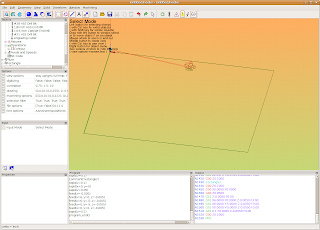I opened up the output_file_name.ngc file in gedit and then pasted in the code created in HeeksCNC. Here it is in emc2/axis:Here is a finished piece, mounted to a nice mahogany board:## Thursday, May 20, 2010

### Running Python Scripts with the 'Program' Window

HeeksCNC has a unique feature that I really like: all operations create python code that gets processed to make G-code. In the 'Window' menu check the 'Program' option. While you are creating operations and G-code, look at what is happening in the 'Program' window.You can manipulate the python code in the Program window to do all sorts of interesting things. I think that this feature in HeeksCNC gives it a lot of power, similar to APT360. I am very familiar with APT programming and like it a lot, but I really prefer python and the HeeksCNC approach.

The code from the Program window can be edited and used in the window itself. Simply edit the code and then press the 'Py+Go' (Run Python Script) button. Don't just press the 'Go' button, because it will populate the Program window with the normal python code that comes from the operations in HeeksCNC.

You can also copy and edit the python code in your favorite text editor, if you like. I do that because it's what I am familiar with.

The python code can also be run in a stand alone mode- outside of HeeksCNC, if you are careful to fix up any path problems. I sometimes run the python scripts this way, if I have my geometry set and just want to try different variables out with the code, ie different depths of cut.

One simple thing that you can do with the code is to use an operation over and over again with different size tool diameters or different depths. 'if' or 'while' loops make this sort of thing pretty easy to deal with.
In the next screen shot, I took the kurve_funcs.py module and copied/edited it and saved it as rough_funcs.py (I commented out #rapid(z = mat_depth + incremental_rapid_height) on line 229). I simply wanted to keep the tool down in the work for my next trick: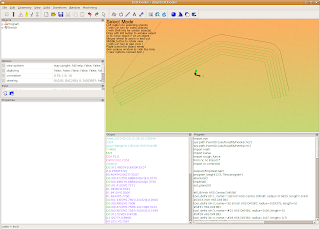Here is my python code that I pasted into the Program window:

import sys
sys.path.insert(0,'/usr/local/lib/heekscnc/')
sys.path.insert(0,'/usr/local/lib/heekscnc')
import math
import kurve
import rough_funcs
from nc.nc import *
import nc.centroid1

output('/tmp/test.tap')
program_begin(123, 'Test program')
absolute()
imperial()
set_plane(0)

#(1/8 inch HSS Centre Drill Bit)
tool_defn( id=1, name='1/8 inch HSS Centre Drill Bit', radius=0.0625, length=0.94)
#(3/16 inch HSS Drill Bit)
tool_defn( id=2, name='3/16 inch HSS Drill Bit', radius=0.09375, length=5)
#(#31 HSS Drill Bit)
tool_defn( id=3, name='#31 HSS Drill Bit', radius=0.06, length=5)
#(#28 HSS Drill Bit)
tool_defn( id=4, name='#28 HSS Drill Bit', radius=0.07, length=0.7)
#(1/8 inch HSS End Mill)
tool_defn( id=5, name='1/8 inch HSS End Mill', radius=0.0625, length=2.5)
#(1/16 inch Carbide End Mill)
tool_defn( id=6, name='1/16 inch Carbide End Mill', radius=0.03125, length=0.3125)
#(#21 HSS Drill Bit)
tool_defn( id=7, name='#21 HSS Drill Bit', radius=0.0795, length=5)
#(engraving cutter)
tool_defn( id=20, name='engraving cutter', radius=0.0625, length=0.625)
comment('G54')
workplane(1)
comment('tool change to 1/8 inch HSS End Mill')
tool_change( id=5)
spindle(7000)
feedrate_hv(33.07086614, 3.937007874)
flush_nc()
clearance = float(0.1968503937)
rapid_down_to_height = float(0.07874015748)
start_depth = float(0)
step_down = float(0.25)
final_depth = float(-0.25)
roll_on = 'auto'
roll_off = 'auto'
tag = None

roll_radius = float(0)
offset_extra = 0
comment('Sketch')
k1 = kurve.new()
kurve.add_point(k1, 0, -1.732283465, -0.7086614173, 0.0, 0.0)
kurve.add_point(k1, 0, 0.157480315, 0.9448818898, 0.0, 0.0)
kurve.add_point(k1, -1, 0.4724409449, 1.062992126, 0.4718257874, 0.5856299213)
kurve.add_point(k1, 0, 1.417322835, 1.102362205, 0.0, 0.0)
kurve.add_point(k1, -1, 1.732283465, 0.9448818898, 1.432465172, 0.7389460933)
kurve.add_point(k1, 0, 2.125984252, 0.07874015748, 0.0, 0.0)

k2 = kurve.new()
kurve.add_point(k2, 0, 2.125984252, 0.07874015748, 0.0, 0.0)
kurve.add_point(k2, 0, 1.732283465, 0.9448818898, 0.0, 0.0)
kurve.add_point(k2, 1, 1.417322835, 1.102362205, 1.432465172, 0.7389460933)
kurve.add_point(k2, 0, 0.4724409449, 1.062992126, 0.0, 0.0)
kurve.add_point(k2, 1, 0.157480315, 0.9448818898, 0.4718257874, 0.5856299213)
kurve.add_point(k2, 0, -1.732283465, -0.7086614173, 0.0, 0.0)

tool_diameter = float(0.75)
rough_funcs.profile(k1, 'right', tool_diameter/2, offset_extra, roll_radius, roll_on, roll_off, tag, rapid_down_to_height, start_depth, step_down, final_depth)

tool_diameter = float(0.625)
rough_funcs.profile(k2, 'left', tool_diameter/2, offset_extra, roll_radius, roll_on, roll_off, tag, rapid_down_to_height, start_depth, step_down, final_depth)

tool_diameter = float(0.5)
rough_funcs.profile(k1, 'right', tool_diameter/2, offset_extra, roll_radius, roll_on, roll_off, tag, rapid_down_to_height, start_depth, step_down, final_depth)

tool_diameter = float(0.375)
rough_funcs.profile(k2, 'left', tool_diameter/2, offset_extra, roll_radius, roll_on, roll_off, tag, rapid_down_to_height, start_depth, step_down, final_depth)

tool_diameter = float(0.25)
rough_funcs.profile(k1, 'right', tool_diameter/2, offset_extra, roll_radius, roll_on, roll_off, tag, rapid_down_to_height, start_depth, step_down, final_depth)

tool_diameter = float(0.125)
rough_funcs.profile(k2, 'left', tool_diameter/2, offset_extra, roll_radius, roll_on, roll_off, tag, rapid_down_to_height, start_depth, step_down, final_depth)

rapid(z = clearance)

program_end()

## Sunday, May 16, 2010

### Tooltable in HeeksCNC

HeeksCNC has a nice tooltable feature in the Objects Tree.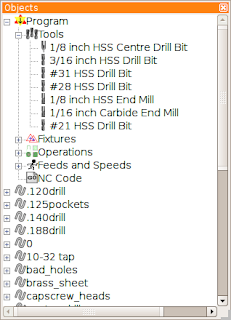You can edit the tools and export the tooltable and later re import it. I set up a default tool table named 'default.tooltable' that loads every time I open HeeksCNC.

This tooltable corresponds to the tools that I actually have loaded in my milling machine (or the values for the height offsets I have stored in it, since I don't have an automatic tool changer).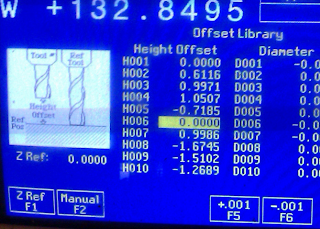Here is my tool changing 'carousel':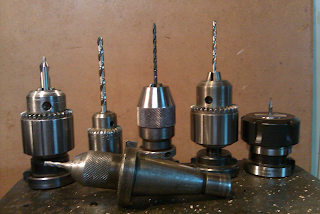## Sunday, May 9, 2010

### importing into HeeksCADQcad drawings import into HeeksCAD with no problems. In the 'Objects' panel in the upper left area, you can see that all the layers are listed. If you click on one of the layers, you will see some of it's properties and can choose to make it not visible. This is handy for working on a CNC program with HeeksCNC.

### sketching and importingI'm using Qcad to do all the sketching on my latest project. I have gotten used to how Qcad works and it's not too bad. One thing that I haven't figured out is if Qcad can import other files. So far, I don't think that it can.

## Saturday, May 8, 2010

### HeeksCNC Postprocessing

I've been working with HeeksCNC over the last few weeks on a project that I'm going to mill out of brass. Since I have a Centroid M40 control on my CNC milling machine, I need a proper postprocessor for it. On Friday, I created one and did some editing to Dan Heeks' iso_modal post. Now I think I have things the way that I want them.
Anyone interested can look at the files here:
http://code.google.com/p/heekscnc/source/browse/#svn/trunk/nc
Look at centroid1.py, centroid1_read.py and machines.txt to see what I did.